## Saturday, April 29, 2017

When Gurobi returns:

 Barrier performed 80 iterations in 18.11 secondsSub-optimal termination - objective 1.51290046e+02

 QP status(13): unknown

and does not return the solution:

 **** MODEL STATUS      14 No Solution Returned

This has been fixed for the next release.

### VirtualBox and sharing folders

With a brand new VirtualBox and a new Ubuntu desktop (as client on a Windows machine), I had troubles getting the folder sharing to work. The mount command failed with some rather obscure messages. The solution was to install: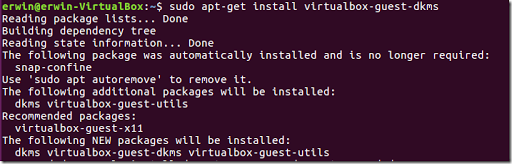##### References
1. HOWTO: Using Shared Folders, https://forums.virtualbox.org/viewtopic.php?f=29&t=15868&sid=48768b562c0cf8d0f002e47277f786fb

## Matrix vs Data Frame

In R there are obvious ways to store a rectangular grid of numbers: a matrix of a data frame. A data frame can handle different types in different columns, so it is richer than a matrix which has a single type. Internally a matrix is just a vector with a dimension atribute.

A dataframe can be accessed as if it is a matrix using the notation df[i,j].

When we want to access a large number of elements a[i,j] (unvectorized), there is a difference in timing however.

Lets first create a 100x100 matrix.

# create a 100 x 100 matrix with random numbers
n <- 100
m <- 100
a <- matrix(runif(n*m,0,100),nrow=m,ncol=n)
str(a)
##  num [1:100, 1:100] 85.1 17.7 34.9 54.2 51 ...

Now copy the data into a data frame:

df <- as.data.frame(a)
tibble::glimpse(df[,1:10])
## Observations: 100
## Variables: 10
## $V1 <dbl> 85.065933, 17.728731, 34.860332, 54.248920, 50.977486, 99.... ##$ V2  <dbl> 5.813586, 56.404924, 41.151549, 32.029510, 40.857083, 81.8...
## $V3 <dbl> 4.452198, 34.878083, 34.398440, 90.276525, 26.451508, 48.9... ##$ V4  <dbl> 43.496058, 95.162642, 65.250058, 83.941005, 55.507790, 17....
## $V5 <dbl> 58.70423852, 39.08486762, 73.75686767, 51.61834429, 72.739... ##$ V6  <dbl> 34.55152, 36.22242, 12.87432, 74.17734, 32.98368, 93.05312...
## $V7 <dbl> 96.112254, 55.291468, 8.653270, 28.452267, 18.926653, 80.6... ##$ V8  <dbl> 45.4440507, 20.2528389, 98.3861469, 90.8775842, 14.8820597...
## $V9 <dbl> 3.2462605, 10.2364068, 90.3974374, 5.3393112, 41.1585281, ... ##$ V10 <dbl> 39.686012, 74.177476, 67.095580, 96.879985, 16.446060, 97....

Here we compare the memory use. They are roughly the same.

pryr::object_size(a)
pryr::object_size(df)
## 80.2 kB
## 91 kB

Let’s create a function that randomly access one million elements. To be able to check that we do the same thing we sum these elements.

K <- 1e6
rowi <- sample(n,size=K,replace=T)
colj <- sample(m,size=K,replace=T)
f <- function(a) {
s <- 0
for(k in 1:K) {
s <- s + a[rowi[k],colj[k]]
}
return(s)
}
# same result: we compute the same thing
f(a)
f(df)
##  49894303
##  49894303

The results of calling f(a) and f(df) are the same, but the data frame version takes much more time:

system.time(f(a))
system.time(f(df))
##    user  system elapsed
##    2.74    0.00    2.75
##    user  system elapsed
##   50.71    0.02   50.81

## Matrix vs Data Frame

In R there are obvious ways to store a rectangular grid of numbers: a matrix of a data frame. A data frame can handle different types in different columns, so it is richer than a matrix which has a single type. Internally a matrix is just a vector with a dimension atribute.

A data frame can be accessed as if it is a matrix using the notation df[i,j].

When we want to access a large number of elements a[i,j] (unvectorized), there is a difference in timing however.

Let's first create a 100 x 100 matrix.

# create a 100 x 100 matrix with random numbers
m <- 100
n <- 100
a <- matrix(runif(m*n,0,100),nrow=m,ncol=n)
str
(a)

##  num [1:100, 1:100] 16.6 43.1 86.7 44.4 11.1 ...

Now copy the data into a data frame using as.data.frame(a). I could have used data.frame(a) instead (that would yield different column names X1,X2,...).

df <- as.data.frame(a)
df[1:5,1:5
]

##         V1       V2       V3          V4       V5
## 1 16.63825 37.27615 91.70009 31.16568013 60.35773
## 2 43.07365 26.23530 45.98191 27.83072027 94.46351
## 3 86.72209 14.70443 55.62293 51.58801596 83.38001
## 4 44.37759 93.33136 41.71945  0.06141574 75.64287
## 5 11.13246 14.10785 75.97597 51.34233858 54.39535

Here we compare the memory use. They are roughly the same.

pryr::object_size(a)
pryr::object_size
(df)

## 80.2 kB
## 91 kB

Let's create a function that randomly accesses one million elements. To be able to check that we do the same thing we sum these elements.

K <- 1e6
rowi <- sample(m,size=K,replace=T)
colj <- sample(n,size=K,replace=T)
f <- function(a) {
s <- 0
for(k in 1:K) {
s <- s + a[rowi[k],colj[k]]
}
return(s)
}
# same result: we compute the same thing
f(a)
f
(df)

##  49990017
##  49990017

The results of calling f(a) and f(df) are the same, but the data frame version takes much more time:

system.time(f(a))
system.time(f
(df))

##    user  system elapsed
##    3.18    0.00    3.20
##    user  system elapsed
##   58.39    0.07   59.31

Note: don't try this function on a Data Table. The behavior and rules of indexing on a Data Table are slightly different. Although we can use:

dt <- data.table(df)
dt[1,2
]

##          V2
## 1: 37.27615

the indexing as used in function f() is not identical to what we are used to when working with data frames and matrices.

## Thursday, April 27, 2017

### Echos from the past: MS Solver Foundation

In a recent post (1) a nice introduction into Microsoft Solver Foundation is provided. Unfortunately Solver Foundation has been discontinued a while ago; the last version was 3.1 in 2012.

The author is from Ukraine. I think there was a fellow countryman who was once very active in the Optimization arena with his Python tools. Dmitrey Kroshko had a fairly active website (openopt.org). I have no idea what happened to Dmitrey or OpenOpt.

##### References
1. Juri Krivoruchko, Solving optimization problems with Microsoft Solver Foundation, https://www.codeproject.com/Articles/1183168/Solving-optimization-problems-with-Microsoft-Solve
2. Solver Foundation Enterprise, http://yetanothermathprogrammingconsultant.blogspot.com/2012/05/solver-foundation-enterprise.html
3. https://pypi.python.org/pypi/openopt

## Tuesday, April 25, 2017

### Excel Power Query and CSV files

Power Query (or Get and Transform as it is called now) has some interesting tools to import a bunch of CSV files. Here are some notes.

#### Line Endings

Here is a small set of CSV files: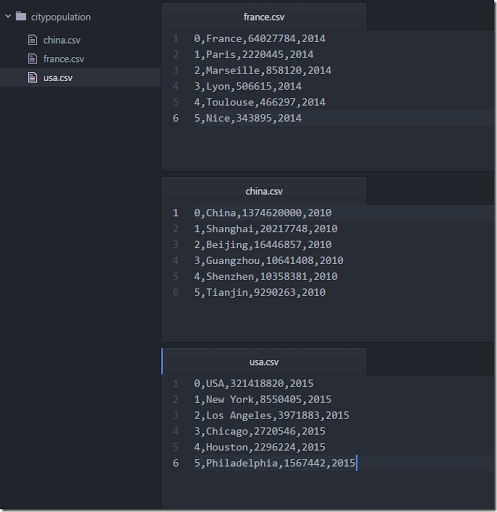When we load this with New Query|From File|From Folder and Edit we see: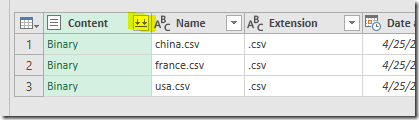Pressing the button with the arrows in the Content column leads to: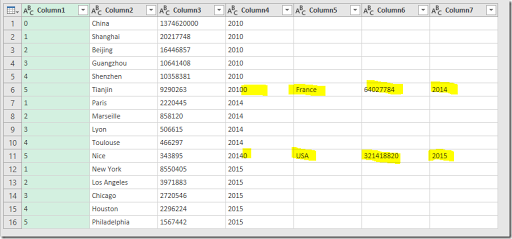The reason for this behavior is: the last line of each CSV file does not have a proper line ending. Most CSV readers are forgiving in this respect, Excel (in this case) is not.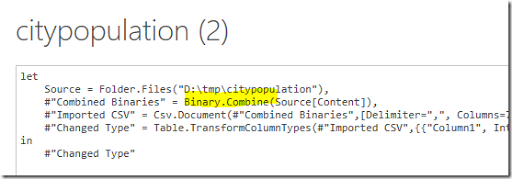The auto-generated script (shown above) is combining the content of the CSV files using the Binary.Combine function. I don’t know an easy way to fix this in the generated import script so instead I fixed the CSV files themselves, so we can concentrate on the handling of headers.

If we have headers in the CSV files, as in: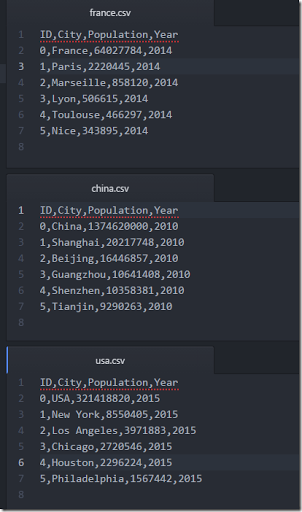we can promote the first record of the combined data to form our column names. Unfortunately, there will be remaining headers in the records: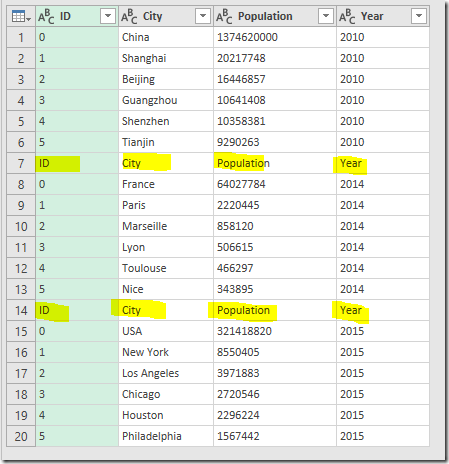These header records can be filtered out explicitly. After applying the row filter and fixing up the column types, we have: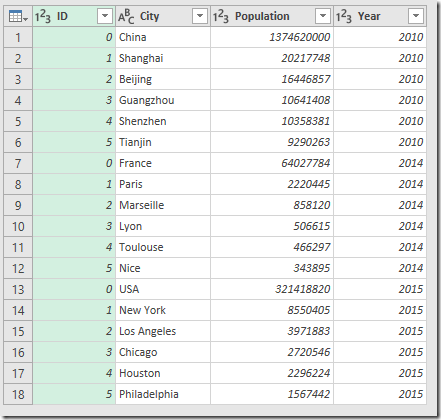I changed the types of column ID, Population and Year to “whole number”. Note: it is not always clear if an ID should be a numeric value. There are good reasons to keep it text so we cannot apply numeric operations on this column.

The generated script looks like:

 let    Source = Folder.Files("D:\tmp\citypopulation"),    #"Combined Binaries" = Binary.Combine(Source[Content]),    #"Imported CSV" = Csv.Document(#"Combined Binaries",[Delimiter=",", Columns=4, Encoding=1252, QuoteStyle=QuoteStyle.None]),    #"Promoted Headers" = Table.PromoteHeaders(#"Imported CSV"),    #"Filtered Rows" = Table.SelectRows(#"Promoted Headers", each [ID] <> "ID"),    #"Changed Type" = Table.TransformColumnTypes(#"Filtered Rows",{{"Population", Int64.Type}, {"Year", Int64.Type}, {"ID", Int64.Type}})in    #"Changed Type"

#### Filename as column

In this case we also want to the country name as extra column in the final table. This name can be deduced from the file name. This type of operation is not unusual when working with CSV files. The following script will do the job:

 let    Source = Folder.Files("D:\tmp\citypopulation"),    #"Added Column 1" = Table.AddColumn(Source, "CountryName", each Text.Replace([Name],[Extension],"")),    #"Added Column 2" = Table.AddColumn(#"Added Column 1", "Data", each Table.PromoteHeaders(Csv.Document([Content], [Delimiter=",", Columns=4, Encoding=1252, QuoteStyle=QuoteStyle.None]))),    #"Select Columns" = Table.SelectColumns(#"Added Column 2",{"CountryName", "Data"}),    Expanded = Table.ExpandTableColumn(#"Select Columns", "Data", {"ID", "City", "Population", "Year"}, {"ID", "City", "Population", "Year"}),    #"Changed Type" = Table.TransformColumnTypes(Expanded,{{"ID", Int64.Type}, {"Population", Int64.Type}, {"Year", Int64.Type}})    in    #"Changed Type"

Basically the algorithm is:

2. Added Column 1: add column “CountryName” by dropping the extension from the filename (i.e. “china.csv” becomes “china”).
3. Added Column 2: add a column “Data” that will contain the content of each CSV file (a so-called table column).
4. Select Columns: keep only these two columns.
5. Expanded: Expand the “Data” table column so that columns inside the “Data” tables become columns of our main table. This is a pretty powerful function.
6. Changed Type: fix up the column types.

This results in: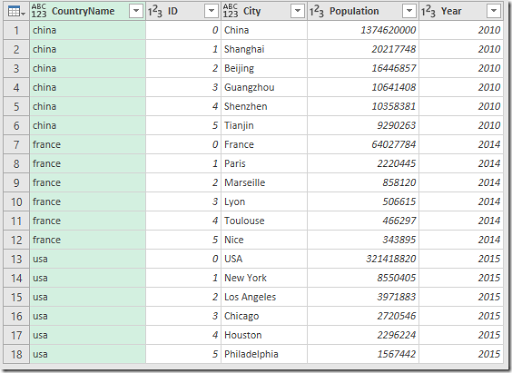With this script we actually no longer need to worry about line endings of the last record in each file: the Csv.Document function (inside the “Added Column 2” step) will take care of that.

#### Extract only *.CSV files from directory

If the directory contains other files than *.csv files we can apply a row filter on the source table we get from the Folder.Files function. Let’s also make sure we handle both *.csv and *.CSV files:

 let    Source = Folder.Files("D:\tmp\citypopulation"),    CsvFiles = Table.SelectRows(Source, each Text.Lower([Extension]) = ".csv"),    . . .

or if we don’t want to introduce an extra step:

 let    Source = Table.SelectRows(Folder.Files("D:\tmp\citypopulation"), each Text.Lower([Extension]) = ".csv"),    . . .

Note that it is not allowed to use something like: Folder.Files("D:\tmp\citypopulation\*.csv").

#### The M Language

To work with the scripts it is useful to know a little bit about the M language, see (3) and (4). Microsoft is very good in creating texts with a somewhat high PR level. The next sentences are from (4):

The Power Query Formula Language is a powerful query language optimized for building queries that mashup data. It's a functional, case sensitive language similar to F#, which can be used with Power Query in Excel, Get & Transform in Excel 2016, and Power BI Desktop.

For computer language theorists: Power Query is a mostly pure, higher-order, dynamically typed, partially lazy, functional language. You can create a variety of data mashup queries from simple to advanced. As with any programming language, you will need some intermediate programming experience for more advanced scenarios.

#### References

1. Data is from https://www.citypopulation.de/
2. Imke Feldmann, Power BI: Open multiple CSV files in a folder at once with transactions in Power Query, https://www.youtube.com/watch?v=whu1CsoZn1Q&feature=youtu.be for a slightly different approach regarding the last script
3. Microsoft, Power Query M Reference, https://msdn.microsoft.com/en-us/library/mt211003.aspx
4. Microsoft, Introduction to Power Query (informally known as "M") Formula Language, https://msdn.microsoft.com/en-us/library/mt270235.aspx

### Alternative portfolio mean-variance formulations

In (1) and (2) a portfolio optimization model was used as an example to illustrate a linear reformulation of a QP problem. The problem was stated as:
 \bbox[lightcyan,10px,border:3px solid darkblue]{\begin{align} \min \>& \sum_t w_t^2\\ & w_t = \sum_i r’_{i,t} x_i\\ & \sum_i \mu_i x_i \ge R\\ & \sum_i x_i = 1\\ &x_i\ge 0\end{align}}
Here we denote:
• $$x_i$$ are the optimal (no short) positions (these are the decision variables),
• $$R$$ is the minimum required portfolio return,
• $$r’_{i,t}=r’_{i,t}-\mu_i$$ are the mean-adjusted (historic) returns
• $$w_t$$ are intermediate variables
The more familiar mean-variance model is:
 \bbox[lightcyan,10px,border:3px solid darkblue]{\begin{align} \min&\>x^TQx = \sum_{i,j} q_{i,j} x_i x_j \\ & \sum_i \mu_i x_i \ge R\\ & \sum_i x_i = 1\\ &x_i\ge 0\end{align}}
Here $$Q$$ is the variance-covariance matrix.
These models are essentially the same. Using the fact how $$Q$$ could have been calculated:
 $q_{i,j} = \frac{1}{T} \sum_t (r_{i,t}-\mu_i)(r_{j,t}-\mu_j)$
we can write:
 \begin{align} \sum_{i,j} q_{i,j} x_i x_j &= \frac{1}{T}\sum_{i,j}\sum_t \left(r_{i,t}-\mu_i\right)\left(r_{j,t}-\mu_j\right) x_i x_j\\ &= \frac{1}{T} \sum_t \left(\sum_i r’_{i,t} x_i\right)\left(\sum_j r’_{j,t} x_j\right)\\ &=\frac{1}{T} \sum_t w_t^2 \end{align}
I dropped the factor $$\frac{1}{T}$$ from the model.

This means:
1. These models are indeed identical
2. We have implicitly proven that the covariance matrix is positive semi-definite as $$w_t^2\ge 0$$
3. This formulation can be use to derive a linear alternative by replacing the objective $$\min \sum_t w_t^2$$ by $$\min \sum_t |w_t|$$ (just a different norm).
4. The simpler quadratic form $$\sum_t w_t^2$$ is often easier to solve
5. When $$N>T$$ (number of instruments is larger than the number of time periods – not so unusual as older returns are less interesting), we have less data in the model: $$Q$$ is $$N \times N$$ while $$r’_{i,t}$$ is $$N \times T$$. In this case we “invent” data when forming the covariance matrix.
Note that nowadays it is popular to solve these type of portfolio models as a conic problem (see (3)). Some solvers convert QP problems (and quadratically constrained problems) automatically in an equivalent conic form.
##### References
1. QP as LP: piecewise linear functions, http://yetanothermathprogrammingconsultant.blogspot.com/2017/04/qp-as-lp-piecewise-linear-functions.html
2. QP as LP: cutting planes, http://yetanothermathprogrammingconsultant.blogspot.com/2017/04/qp-as-lp-cutting-planes.html
3. Erling D. Andersen, Joachim Dahl and Henrik A. Friberg, Markowitz portfolio optimization using MOSEK. MOSEK Technical report: TR-2009-2, http://docs.mosek.com/whitepapers/portfolio.pdf

## Monday, April 17, 2017

### MIP model in R using the OMPR package

A small model with data in R is a good opportunity to try out the OMPR modeling tool (2). Also a first time for me to see the solver Symphony (3) in action.

## Problem Description

From (1) (see references below):

I have 4 stores (1,2,3,4) and I can apply 3 treatments (A,B,C) to each of the 4 stores. Each treatment has its own cost and profit.

The matrix is as follows:

Store   Treatment   Cost    Profit
1   A   50  100
1   B   100 200
1   C   75  50
2   A   25  25
2   B   150 0
2   C   50  25
3   A   100 300
3   B   125 250
3   C   75  275
4   A   25  25
4   B   50  75
4   C   75  125

How can I maximize the profit having a constraint on maximum cost in R? In reality, I have a large number of stores and hence cannot be done manually. Each store can get only 1 treatment.

Loading the data into a data frame is easy with read.table:

df<-read.table(text="
Store   Treatment   Cost    Profit
1   A   50  100
1   B   100 200
1   C   75  50
2   A   25  25
2   B   150 0
2   C   50  25
3   A   100 300
3   B   125 250
3   C   75  275
4   A   25  25
4   B   50  75
4   C   75  125
df
##    Store Treatment Cost Profit
## 1      1         A   50    100
## 2      1         B  100    200
## 3      1         C   75     50
## 4      2         A   25     25
## 5      2         B  150      0
## 6      2         C   50     25
## 7      3         A  100    300
## 8      3         B  125    250
## 9      3         C   75    275
## 10     4         A   25     25
## 11     4         B   50     75
## 12     4         C   75    125

## OMPR Model

I am going to try to model this problem with OMPR (2) and solve it with the Symphony MIP solver (3).

First we need a bunch of packages:

library(dplyr)
library(tidyr)
library(ROI)
library(ROI.plugin.symphony)
library(ompr)
library(ompr.roi)

## Extract data

OMPR is using numbers as indices so it makes sense to extract the cost and profit data as matrices. We do this as follows:

# extract some basic data
stores<-unique(df$Store) stores ##  1 2 3 4 treatments <- levels(df$Treatment)
treatments
##  "A" "B" "C"
num_treatments <- length(treatments)
# cost matrix
cost
##     A   B  C
## 1  50 100 75
## 2  25 150 50
## 3 100 125 75
## 4  25  50 75
# profit matrix
profit
##     A   B   C
## 1 100 200  50
## 2  25   0  25
## 3 300 250 275
## 4  25  75 125
# maximum cost allowed
max_cost <- 300

## MIP Model

The OMPR package allows us to specify linear equations algebraically. Most LP/MIP solvers in R use a matrix notation, which often is more difficult to use. Here we go:

m <- MIPModel() %>%
add_constraint(sum_expr(cost[i,j]*x[i,j], i=stores, j=1:num_treatments) <= max_cost) %>%
set_objective(sum_expr(profit[i,j]*x[i,j], i=stores, j=1:num_treatments),"max") %>%
solve_model(with_ROI(solver = "symphony",verbosity=1))
##
## Starting Preprocessing...
## Preprocessing finished...
##       with no modifications...
## Problem has
##   5 constraints
##   12 variables
##   24 nonzero coefficients
##
## Total Presolve Time: 0.000000...
##
## Solving...
##
## granularity set at 25.000000
## solving root lp relaxation
## The LP value is: -650.000 [0,5]
##
##
## ****** Found Better Feasible Solution !
## ****** Cost: -650.000000
##
##
## ****************************************************
## * Optimal Solution Found                           *
## * Now displaying stats and best solution found...  *
## ****************************************************
##
## ====================== Misc Timing =========================
##   Problem IO        0.000
## ======================= CP Timing ===========================
##   Cut Pool                  0.000
## ====================== LP/CG Timing =========================
##   LP Solution Time          0.000
##   LP Setup Time             0.000
##   Variable Fixing           0.000
##   Pricing                   0.000
##   Strong Branching          0.000
##   Separation                0.000
##   Primal Heuristics         0.000
##   Communication             0.000
##   Total User Time              0.000
##   Total Wallclock Time         0.000
##
## ====================== Statistics =========================
## Number of created nodes :       1
## Number of analyzed nodes:       1
## Depth of tree:                  0
## Size of the tree:               1
## Number of solutions found:      1
## Number of solutions in pool:    1
## Number of Chains:               1
## Number of Diving Halts:         0
## Number of cuts in cut pool:     0
## Lower Bound in Root:            -650.000
##
## ======================= LP Solver =========================
## Number of times LP solver called:                 1
## Number of calls from feasibility pump:            0
## Number of calls from strong branching:            0
## Number of solutions found by LP solve:            1
## Number of bounds changed by strong branching:     0
## Number of nodes pruned by strong branching:       0
## Number of bounds changed by branching presolver:  0
## Number of nodes pruned by branching presolver:    0
##
## ==================== Primal Heuristics ====================
##                              Time      #Called   #Solutions
## Rounding I                   0.00
## Rounding II                  0.00
## Diving                       0.00
## Feasibility Pump             0.00
## Local Search                 0.00            1            0
## Restricted Search            0.00
## Rins Search                  0.00
## Local Branching              0.00
##
## =========================== Cuts ==========================
## Accepted:                         0
## Deleted from LPs:                 0
## Removed because of bad coeffs:    0
## Removed because of duplicacy:     0
## Insufficiently violated:          0
## In root:                          0
##
## Time in cut generation:              0.00
## Time in checking quality and adding: 0.00
##
##                    Time     #Called     In Root       Total
## Gomory             0.00
## Knapsack           0.00
## Clique             0.00
## Probing            0.00
## Flowcover          0.00
## Twomir             0.00
## Oddhole            0.00
## Mir                0.00
## Rounding           0.00
## LandP-I            0.00
## LandP-II           0.00
## Redsplit           0.00
##
## ===========================================================
## Solution Found: Node 0, Level 0
## Solution Cost: -650.0000000000
## +++++++++++++++++++++++++++++++++++++++++++++++++++
## User indices and values of nonzeros in the solution
## +++++++++++++++++++++++++++++++++++++++++++++++++++
##       1 1.0000000000
##       2 1.0000000000
##       4 1.0000000000
##      11 1.0000000000
## 

## Solution

A “raw" solution can be generated using:

get_solution(m,x[i, j]) %>%
filter(value > 0)
##   variable i j value
## 1        x 2 1     1
## 2        x 3 1     1
## 3        x 1 2     1
## 4        x 4 3     1

We want to clean this up a bit:

cat("Status:",solver_status(m),"\n")
cat("Objective:",objective_value(m),"\n")
get_solution(m,x[i, j]) %>%
filter(value > 0) %>%
mutate(Treatment = treatments[j],Store = i) %>%
select(Store,Treatment)
## Status: optimal
## Objective: 650
##   Store Treatment
## 1     2         A
## 2     3         A
## 3     1         B
## 4     4         C
##### References
1. The original problem is from: Mixed linear integer optimization - Optimize Profit based on different treatments to all the stores in R, http://stackoverflow.com/questions/43446194/optimize-profit-based-on-different-treatments-to-all-the-stores-in-r
2. Dirk Schumacher, OMPR: R package to model Mixed Integer Linear Programs, https://github.com/dirkschumacher/ompr
3. Symphony MIP Solver: https://projects.coin-or.org/SYMPHONY
4. Paul Rubin, MIP Models in R with OMPR, http://orinanobworld.blogspot.com/2016/11/mip-models-in-r-with-ompr.html

## Thursday, April 13, 2017

### QP as LP: cutting planes

In (1) I described a simple linearization scheme for a QP model we we can solve it as a straight LP. For a simple (convex) quadratic function $$f(x)$$, instead of solving:

 $\min\> f(x)=x^2$

we solve

 \begin{align} \min\>&z\\ &z \ge a_i x + b_i\end{align}

In this post I do things slightly different: instead of adding the linear inequalities ahead of time, we add them one at the time based on the previously found optimal point. This approach is called a cutting plane technique (2).

##### Example: portfolio model

We consider again the simple portfolio model:

 \bbox[lightcyan,10px,border:3px solid darkblue]{\begin{align} \min \>& \sum_t w_t^2\\ & w_t = \sum_i r’_{i,t} x_i\\ & \sum_i \mu_i x_i \ge R\\ & \sum_i x_i = 1\\ &x_i\ge 0\end{align}}

The model is linearized as an LP model:

 \bbox[lightcyan,10px,border:3px solid darkblue]{\begin{align}\min \>& \sum_t z_t\\ &z_t \ge a_{t,k} w_t + b_{t,k} & \text{Added K\times T cuts}\\ & w_t = \sum_i r’_{i,t} x_i\\ & \sum_i \mu_i x_i \ge R\\ & \sum_i x_i = 1\\ &x_i, z_t\ge 0\end{align}}

Initially we start without cuts. Later on, during the Cutting Plane algorithm, we will add linear cuts in each iteration. The algorithm would look like:

1. $$k:=1$$
2. Solve model LP, let $$w^*_t$$ be the optimal values for $$w_t$$.
3. if $$k=$$MAXIT: STOP
4. Add the constraint $$z_t \ge a_{t,k} w_t + b_{t,k}$$ where $$a_{t,k}=2 w^*_t$$ and $$b_{t,k}=-(w^*_t)^2$$. Note that we add one cut for each $$w_t$$ here (our dataset has $$T=717$$ time periods).
5. $$k:=k+1$$
6. go to step 2

Here we stop simply when a certain number of iterations MAXIT has been exceeded. That can be refined by stopping when the objective does not seem to change much anymore. Another optimization could be to only add cuts that are different from the ones added before (for some $$t$$ we may converge quicker than for others).

The algorithm converges very fast:

 ----    118 PARAMETER report2             obj(LP)         w'w iter1               1.02907546iter2   0.21577087  0.46879016iter3   0.33210537  0.48432099iter4   0.37143835  0.41397603iter5   0.40990988  0.41362293iter6   0.41109694  0.41222051iter7   0.41183602  0.41212331iter8   0.41192720  0.41204267iter9   0.41196991  0.41204112iter10  0.41197620  0.41203782

Note that the optimal QP solution has an objective: 0.41202816.

This is pretty good performance especially because the dataset is not very small (it has 717 time periods and 83 stocks).

Here is a picture of the cuts introduced for the first element $$z_1=w_1^2$$: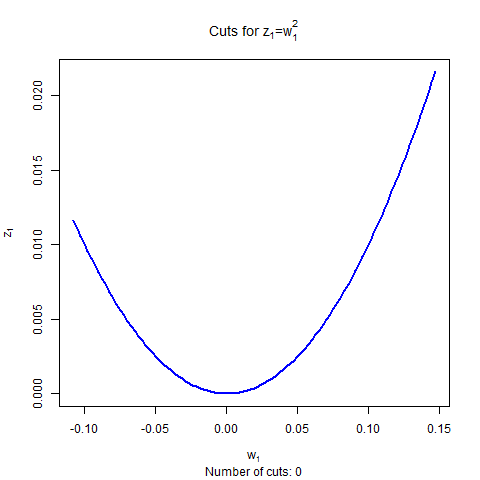##### A combined approach

We can even combine the two methods:

1. Start with a coarse-grained (i.e. cheap) initial set of cuts. In (1) we used $$n=10$$ inequalities per $$w_t$$. For this experiment I reduced this to $$n=5$$.
2. Then apply our cutting plane algorithm. Instead of MAXIT=10 iterations we now do 5 iterations.

This yields even faster convergence:

 ----    142 PARAMETER report3            obj(LP)         w'w iter1  0.32921509  0.41401219iter2  0.40812966  0.41471624iter3  0.41017228  0.41211351iter4  0.41188869  0.41208158iter5  0.41195624  0.41203588
##### References
1. QP as LP: piecewise linear functions, http://yetanothermathprogrammingconsultant.blogspot.com/2017/04/qp-as-lp-piecewise-linear-functions.html
2. J. E. Kelley, Jr. "The Cutting-Plane Method for Solving Convex Programs." J. Soc. Indust. Appl. Math. Vol. 8, No. 4, pp. 703-712, December, 1960.
3. Cardinality Constrained Portfolio Optimization: MIQP without MIQP Solver, http://yetanothermathprogrammingconsultant.blogspot.com/2016/02/cardinality-constrained-portfolio.html. Here this cutting plane method is applied to a MIQP model (not strictly “allowed” as this is no longer convex, but useful as a heuristic).